In this video I show you how to integrate types like this as seen in the examples below.

Although I give you a formula, it is important that you understand the chain rule to fully appreciate the concept rather than rely on the formula.

Formulae: Try and understand how it works rather than learn it.

•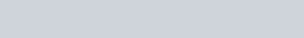Examples: Evaluate the following

1.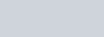2.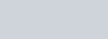3.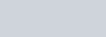Integration : (ax+b)ⁿ type functions : ExamSolutions - youtube Video

Summary Exercise

Examples:

1.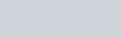2.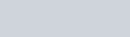3.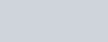Integration : (ax+b)ⁿ type functions - Examples to try : ExamSolutions - youtube Video